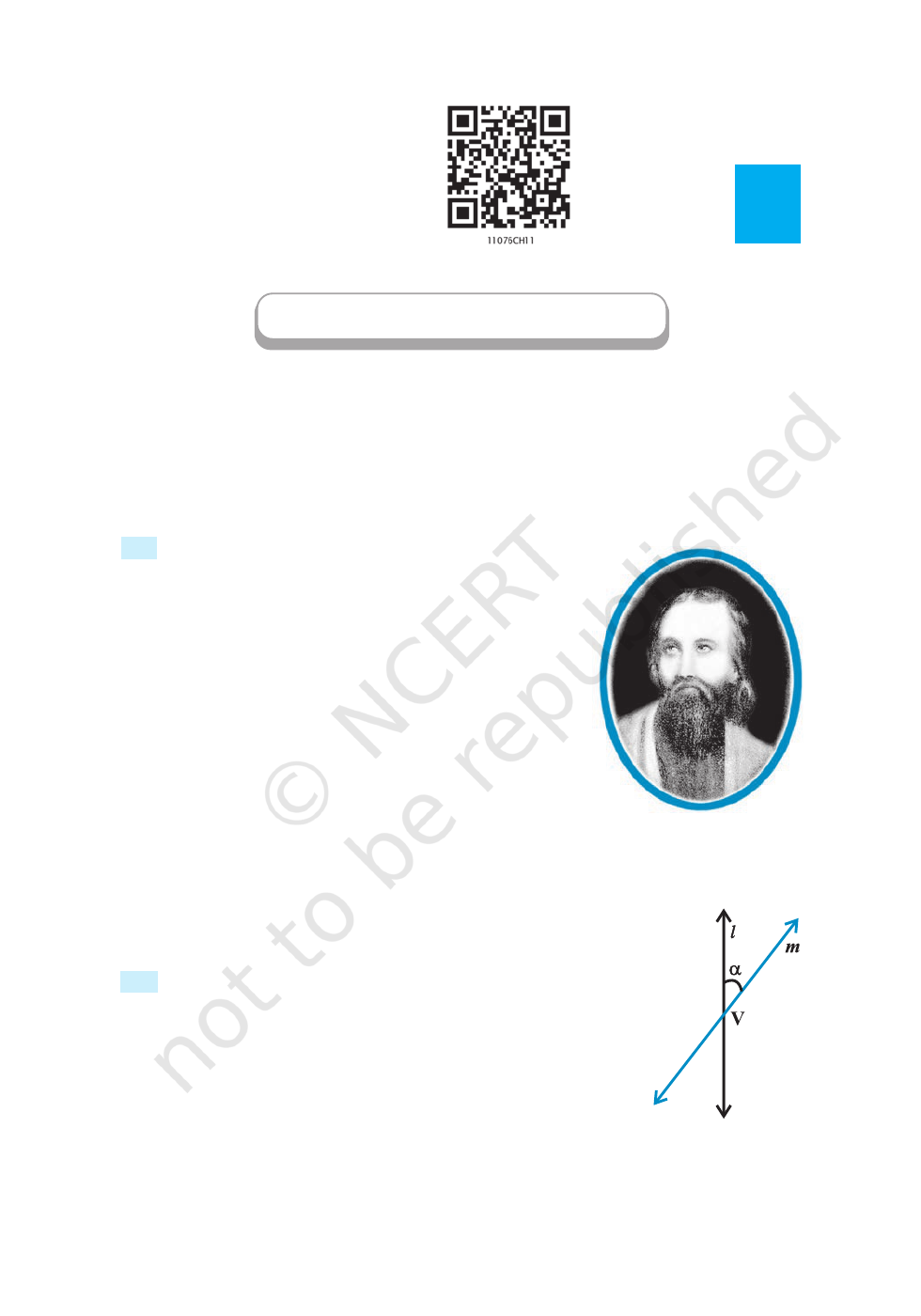vLet the relation of knowledge to real life be very visible to your pupils
and let them understand how by knowledge the world could be
transformed. – BERTRAND RUSSELL v
11.1 Introduction
In the preceding Chapter 10, we have studied various forms
of the equations of a line. In this Chapter, we shall study
about some other curves, viz., circles, ellipses, parabolas
and hyperbolas. The names parabola and hyperbola are
given by Apollonius. These curves are in fact, known as
conic sections or more commonly conics because they
can be obtained as intersections of a plane with a double
napped right circular cone. These curves have a very wide
range of applications in fields such as planetary motion,
design of telescopes and antennas, reflectors in flashlights
and automobile headlights, etc. Now, in the subsequent sections we will see how the
intersection of a plane with a double napped right circular cone
results in different types of curves.
11.2 Sections of a Cone
Let l be a fixed vertical line and m be another line intersecting it at
a fixed point V and inclined to it at an angle α (Fig11.1).
Suppose we rotate the line m around the line l in such a way
that the angle α remains constant. Then the surface generated is
a double-napped right circular hollow cone herein after referred as
Apollonius
(262 B.C. -190 B.C.)
11Chapter
Fig 11. 1
CONIC SECTIONS
2020-21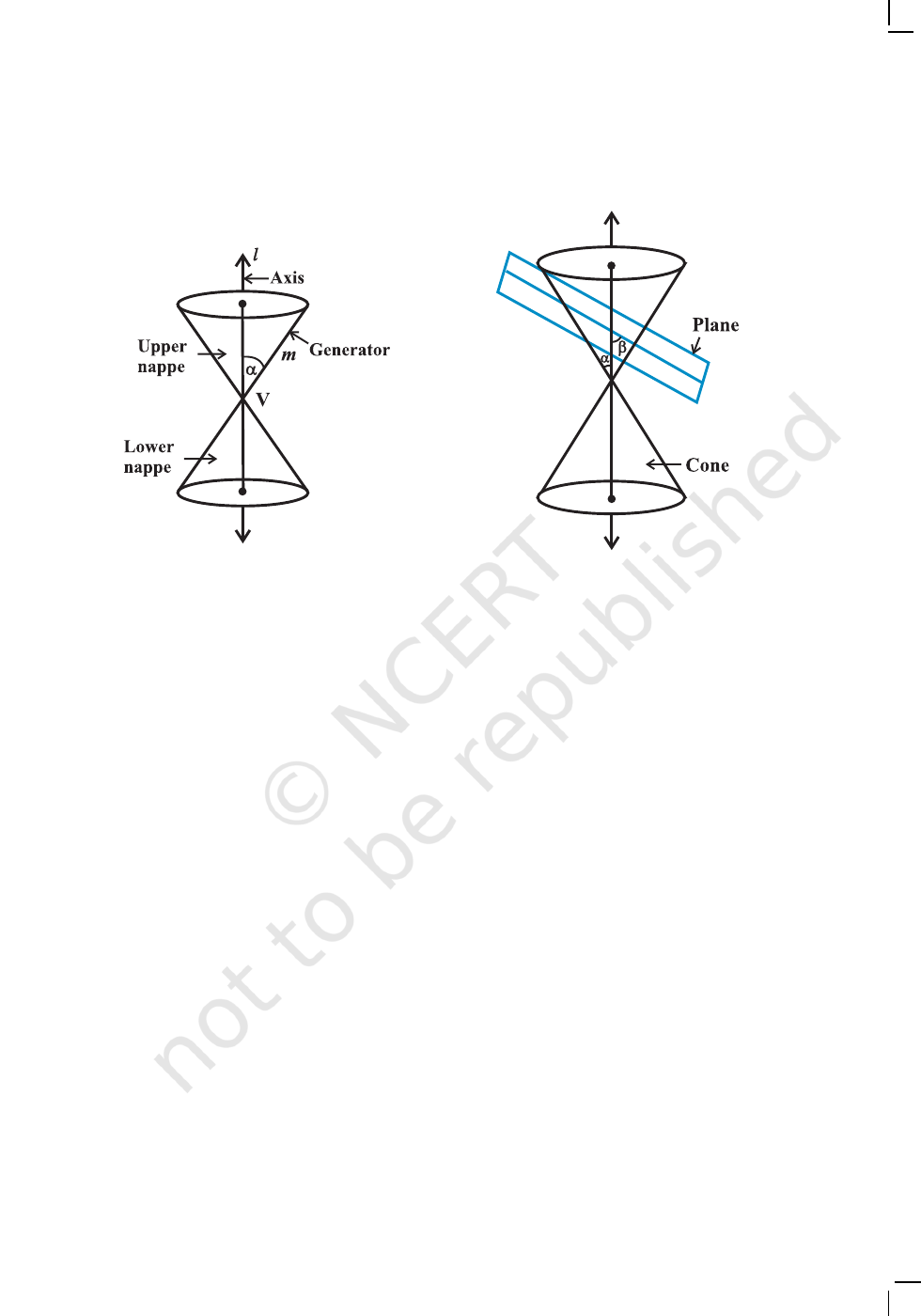CONIC SECTIONS 237
Fig 11. 2
Fig 11. 3
cone and extending indefinitely far in both directions (Fig11.2).
The point V is called the vertex; the line l is the axis of the cone. The rotating line
m is called a generator of the cone. The vertex separates the cone into two parts
called nappes.
If we take the intersection of a plane with a cone, the section so obtained is called
a conic section. Thus, conic sections are the curves obtained by intersecting a right
circular cone by a plane.
We obtain different kinds of conic sections depending on the position of the
intersecting plane with respect to the cone and by the angle made by it with the vertical
axis of the cone. Let β be the angle made by the intersecting plane with the vertical
axis of the cone (Fig11.3).
The intersection of the plane with the cone can take place either at the vertex of
the cone or at any other part of the nappe either below or above the vertex.
11.2.1 Circle, ellipse, parabola and hyperbola When the plane cuts the nappe (other
than the vertex) of the cone, we have the following situations:
(a) When β = 90
o
, the section is a circle (Fig11.4).
(b) When α < β < 90
o
, the section is an ellipse (Fig11.5).
(c) When β = α; the section is a parabola (Fig11.6).
(In each of the above three situations, the plane cuts entirely across one nappe of
the cone).
(d) When 0 β < α; the plane cuts through both the nappes and the curves of
intersection is a hyperbola (Fig11.7).
2020-21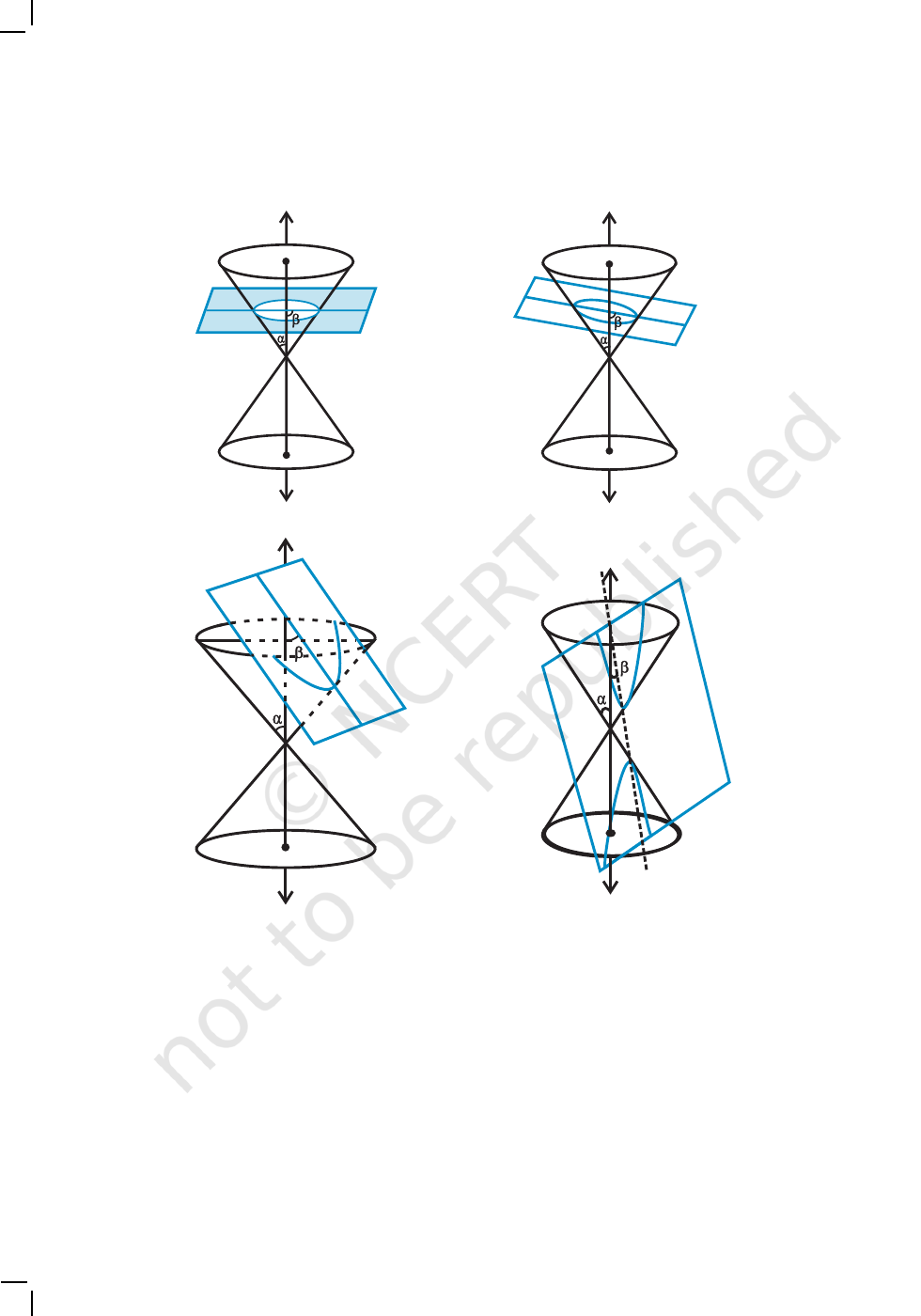238 MATHEMATICS
Fig 11. 4
11.2.2 Degenerated conic sections
When the plane cuts at the vertex of the cone, we have the following different cases:
(a) When α < β 90
o
, then the section is a point (Fig11.8).
(b) When β = α, the plane contains a generator of the cone and the section is a
straight line (Fig11.9).
It is the degenerated case of a parabola.
(c) When 0 β < α, the section is a pair of intersecting straight lines (Fig11.10). It is
the degenerated case of a hyperbola.
Fig 11. 6
Fig 11. 7
Fig 11. 5
2020-21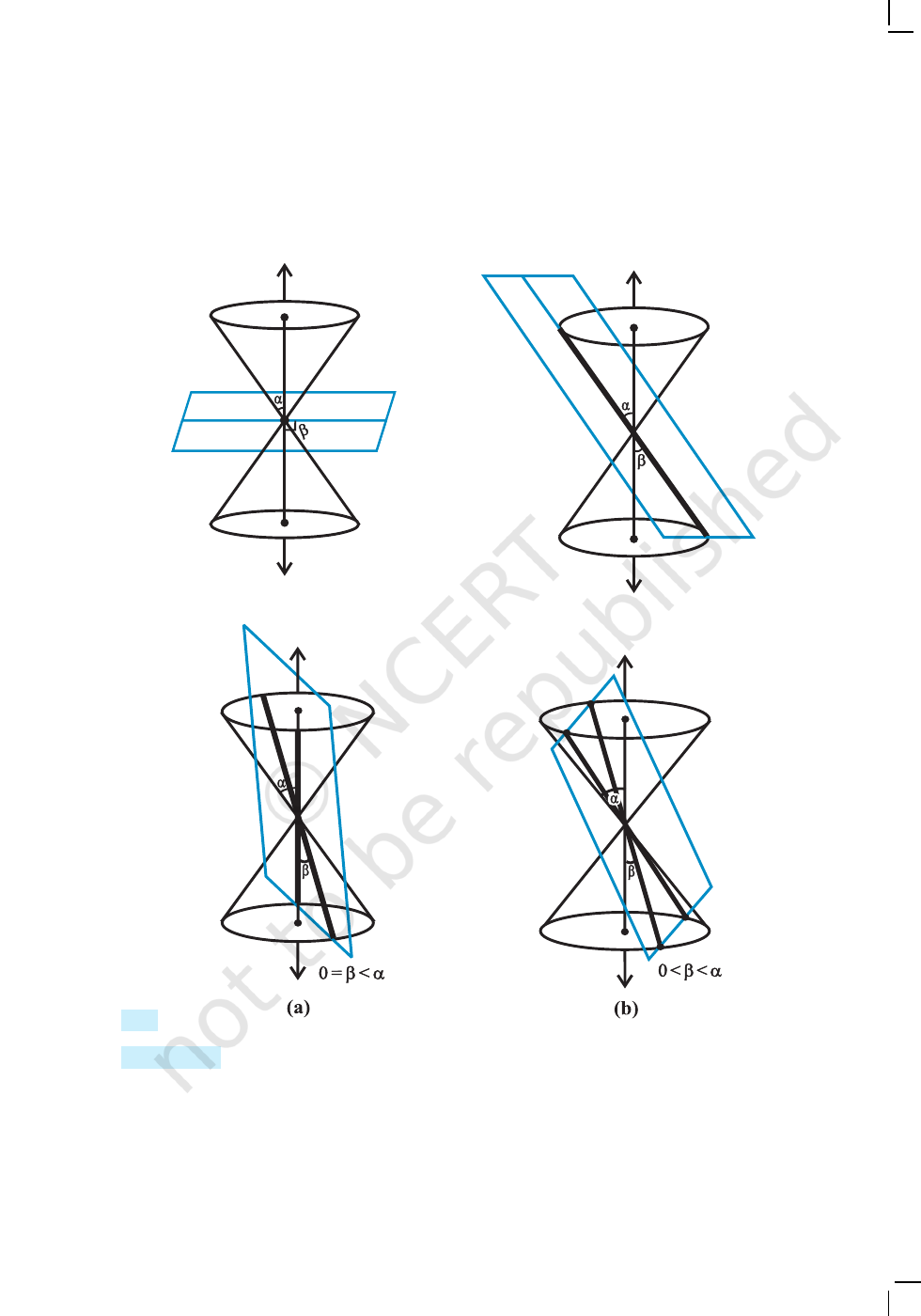CONIC SECTIONS 239
In the following sections, we shall obtain the equations of each of these conic
sections in standard form by defining them based on geometric properties.
Fig 11. 8
Fig 11. 9
Fig 1
1. 10
11.3 Circle
Definition 1 A circle is the set of all points in a plane that are equidistant from a fixed
point in the plane.
The fixed point is called the centre of the circle and the distance from the centre
to a point on the circle is called the radius of the circle (Fig 11.11).
2020-21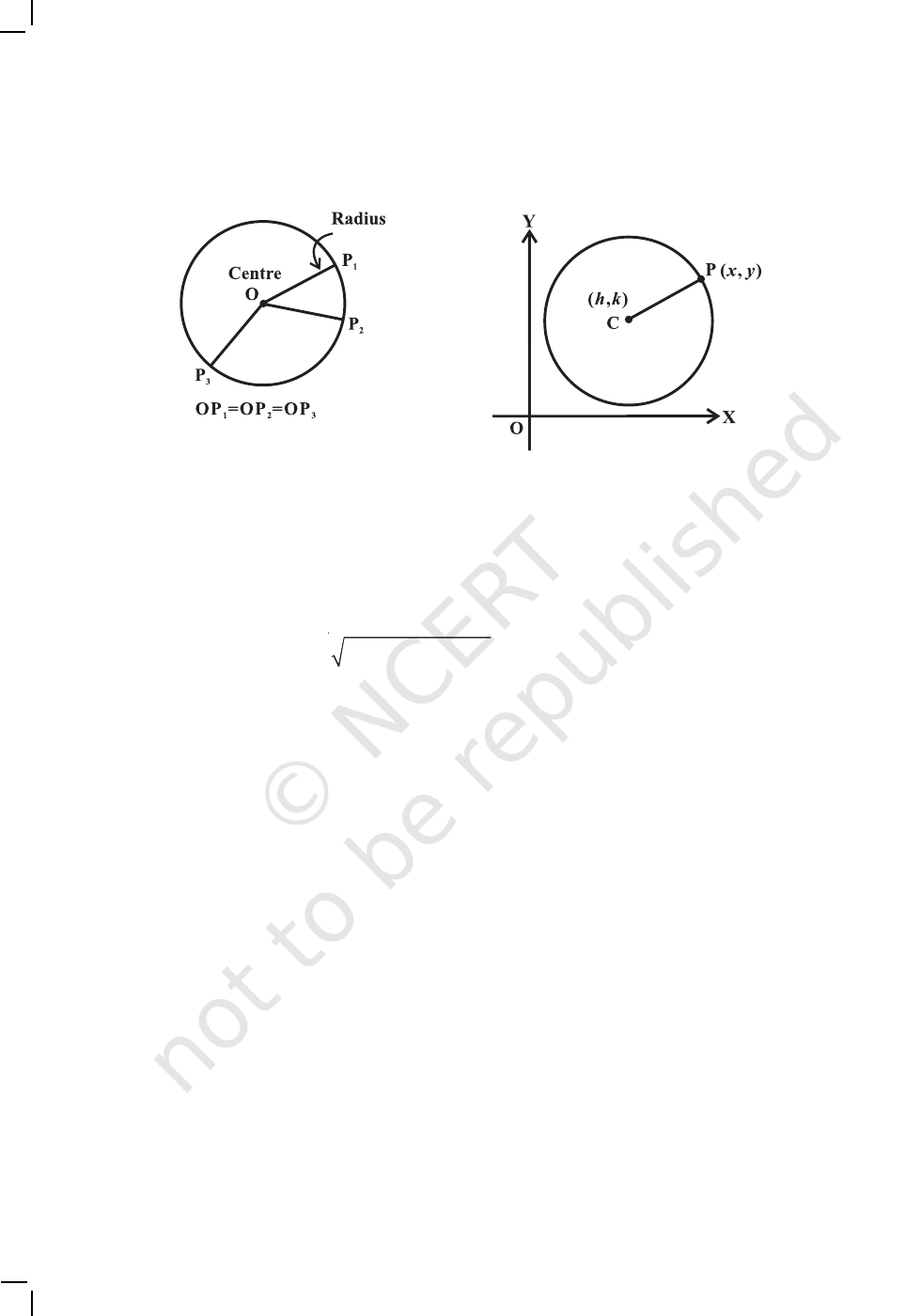240 MATHEMATICS
The equation of the circle is simplest if the centre of the circle is at the origin.
However, we derive below the equation of the circle with a given centre and radius
(Fig 11.12).
Given C (h, k) be the centre and r the radius of circle. Let P(x, y) be any point on
the circle (Fig11.12). Then, by the definition, | CP | = r . By the distance formula,
we have
2 2
( ) ( )
x – h y – k r
+ =
i.e. (x h)
2
+ (yk)
2
= r
2
This is the required equation of the circle with centre at (h,k) and radius r .
Example 1 Find an equation of the circle with centre at (0,0) and radius r.
Solution Here h = k = 0. Therefore, the equation of the circle is x
2
+ y
2
= r
2
.
Example 2 Find the equation of the circle with centre (–3, 2) and radius 4.
Solution Here h = –3, k = 2 and r = 4. Therefore, the equation of the required circle is
(x + 3)
2
+ (y –2)
2
= 16
Example 3 Find the centre and the radius of the circle x
2
+ y
2
+ 8x + 10y – 8 = 0
Solution The given equation is
(x
2
+ 8x) + (y
2
+ 10y) = 8
Now, completing the squares within the parenthesis, we get
(x
2
+ 8x + 16) + (y
2
+ 10y + 25) = 8 + 16 + 25
i.e. (x + 4)
2
+ (y + 5)
2
= 49
i.e. {
x – (– 4)}
2
+ {y – (–5)}
2
= 7
2
Therefore, the given circle has centre at (– 4, –5) and radius 7.
Fig 11. 11
Fig 11. 12
2020-21